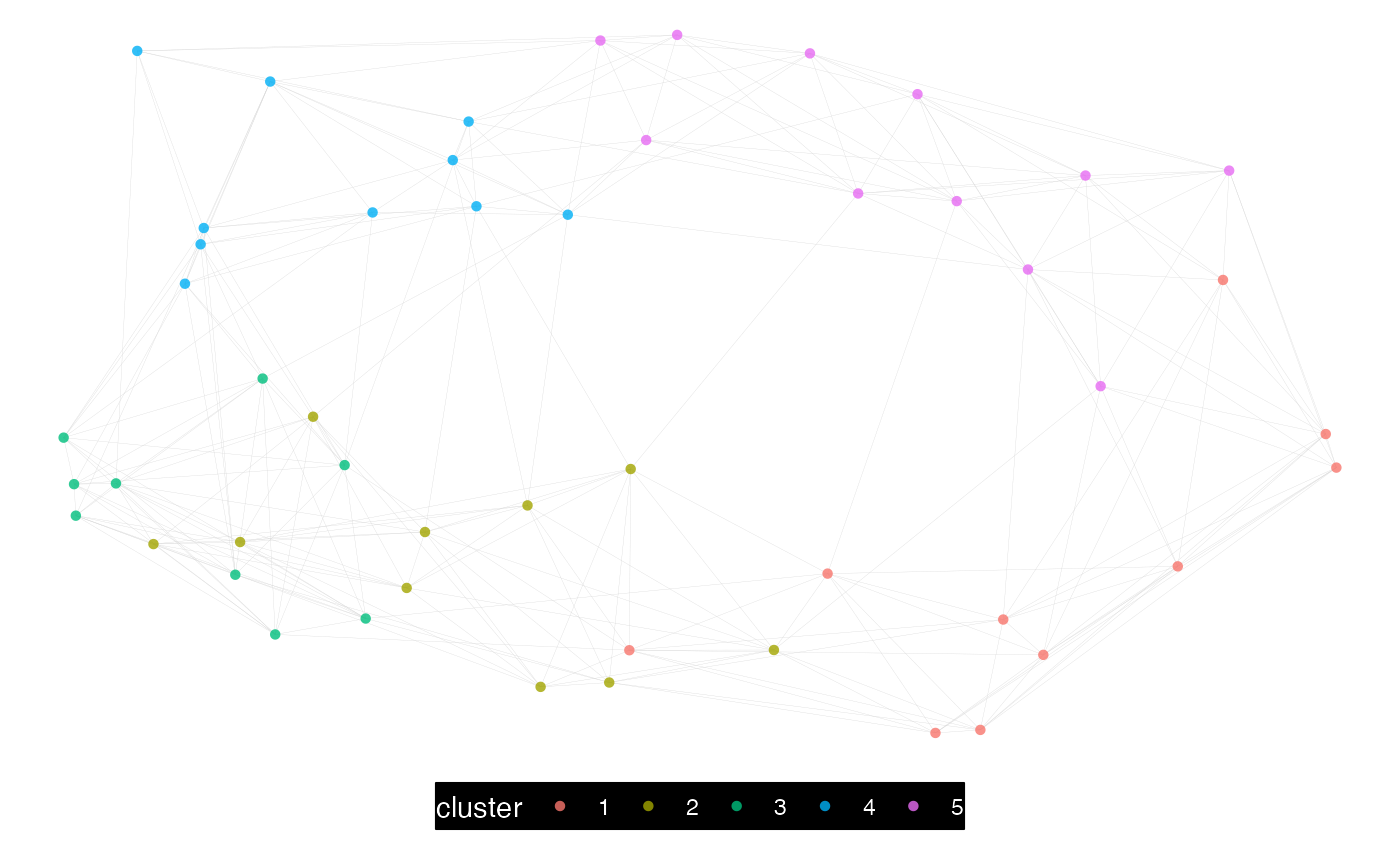Take a P2P network query and implement the Louvain community detection method. The 'igraph' implementation of the Louvain method is used.

network_louvain(
data,
hrvar = "Organization",
bg_fill = "#000000",
font_col = "#FFFFFF",
node_alpha = 0.8,
algorithm = "mds",
path = "network_p2p_louvain",
desc_hrvar = c("Organization", "LevelDesignation", "FunctionType"),
return = "plot-louvain",
size_threshold = 5000
)

## Arguments

data

Data frame containing a person-to-person query.

hrvar

String containing the label for the HR attribute.

bg_fill

String to specify background fill colour.

font_col

String to specify font and link colour.

node_alpha

A numeric value between 0 and 1 to specify the transparency of the nodes. Defaults to 0.7.

algorithm

String to specify the node placement algorithm to be used. Defaults to "mds" for the deterministic multi-dimensional scaling of nodes. See https://rdrr.io/cran/ggraph/man/layout_tbl_graph_igraph.html for a full list of options.

path

File path for saving the PDF output. Defaults to a timestamped path based on current parameters.

desc_hrvar

Character vector of length 3 containing the HR attributes to use when returning the "describe" output. See network_describe().

return

String specifying what output to return. Defaults to "plot-louvain". Valid return options include:

• 'plot-louvain': return a network plot coloured by Louvain communities, saving a PDF to path.

• 'plot-hrvar': return a network plot coloured by HR attribute, saving a PDF to path.

• 'plot-sankey': return a sankey plot combining communities and HR attribute.

• 'table': return a vertex summary table with counts in communities and HR attribute.

• 'data': return a vertex data file that matches vertices with communities and HR attributes.

• 'describe': return a list of data frames which describe each of the identified communities. The first data frame is a summary table of all the communities.

• 'network': return 'igraph' object.

size_threshold

Numeric value representing the maximum number of edges before network_leiden() switches to use a more efficient, but less elegant plotting method (native igraph). Defaults to 5000. Set as 0 to coerce to a fast plotting method every time, and Inf to always use the default plotting method (with 'ggraph').

## Value

See return.

Other Network: external_network_plot(), g2g_data, internal_network_plot(), network_describe(), network_g2g(), network_leiden(), network_p2p(), network_summary(), p2p_data_sim()

## Examples

# Simulate a small person-to-person dataset
p2p_data <- p2p_data_sim(size = 50)

# Return louvain, console, plot
p2p_data %>%
network_louvain(path = NULL,
return = "plot")